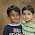## Tuesday, October 14, 2014

### Vectors Parallelogram Law,Triangle Law and Applications

If two vectors are having equal magnitude and certain angle between them , we can find the resultant of the two vectors using the parallelogram law as shown. Using the same concept it can be proved that, if the resultant of two equal and vectors is equal to any one of the vectors then the angle between them is 120°.

Vectors subtraction is similar to that of the vector addition the only differences will be getting an extra negative sign. We can solve all the problems of vectors subtraction using the same concepts of vector addition. All rules like parallelogram law and triangular law can be applied to this concept by taking care of proper signs.

Problem

Let us consider a circular disc of radius R and it is having a translator motion.Find the magnitude and displacement of completion of the have the revolution. Also find the angle made by the resultant.

Lamis Theorem:

This theorem helps in solving some problems vectors. The concept of the theorem and application is solved as explained below. As per this concept, if the vectors are acting and the is in equilibrium, the ratio of the vectors and its opposite sin angle is always constant.

The problem is solved here actually can be solved even with the concept of resolution vectors.

Application of triangular law:

Determination of an external force applied horizontally on the Bob so that the pendulum gets a vertical displacement

Let us consider spherical body having mass m attached to a string of length l two a rigid support as shown. Letters apply a horizontal for F on the spherical body so that it displaces an angle with the vertical. At the instant the spherical body is in a equilibrium position just because there are three forces acting on it as shown.

By resolving the tension into components we can write a equilibrium equations as shown. Here we can calculate force as well as the tension in terms of the weight of the body.

Problem:

Let us  consider two vectors  x and square root of 2 are having some angle between them. Let the resultant of the two vectors is  square root of six and is perpendicular to the vector X . Then find the value of the x and also find the angle between the two vectors ?

In solving this problem we need to take parallelogram law vectors into consideration. It is considered the two vectors is the two sides of the parallelogram. As the resultant is perpendicular to the X the angle between the two vectors shall be more than 90°. The solution is as presented below.

Let us  considers another problem. Let a person is walking 10 m east, then 10 m north and then 10 root 2 m Southwest. Find his final displacement.

In solving this problem we have to treat each of the position as vector. I J and K are the unit vectors along the x-axis y-axis  and z-axis . They choose the direction of the vector. Each vector is represented and the third vector resolved into components as shown. The resultant of all the vectors is simply the vector sum of all of them.

Related Posts

1.hello sir..i m sujata. thank u so much for providing ur handwritten notes..they r amazing...i hav a problem..can u plz tell me that if one of the vector and the resultant is given then how to calculate the 2nd vector..and also the angle?

2.Good very useful

3.thank u so much providing this handwritten notes

4.thank u so much providing handwritten notes